# Random Data Analysis Part 9: Two-Point StatisticsMay 31, 2016 By: Jonathan Dudley

In Part 8, the use of auto and cross-correlations was discussed, which are commonly used to gain an understanding of the relationship between random signals.  For the final post in this series, I will discuss the use of two-point statistics using turbulent velocities or pressures.

Several statistical concepts can be used for the analysis of unsteady quantities of interest,  and in this blog I will focus on unsteady or fluctuating pressure signals. The fluctuating component of the pressure are found from a classical Reynolds decomposition. The following equation gives the fluctuating pressure where the prime represents the fluctuating component of the variable, the overbar represents the mean and a variable with no demarcation represents the instantaneous value.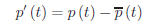The mean takes the traditional definition given in Equation below where the number of independent observations is given by N which is sufficiently large to ensure the mean value has converged.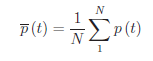The variation of the measured unsteady pressure is studied using the root-mean-square (rms) of the fluctuating variable and is commonly referred to as the standard deviation in statistical literature.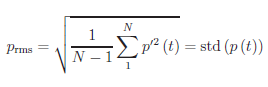The length scale variations in the shear layer are studied by employing a two point spatial cross-correlation on the components of the fluctuating pressure with an origin defined at some fixed point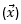with a known separation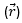. The two point spatial tensor is then given by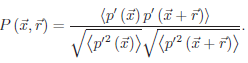where at zero separation, the diagonal elements represent the normal turbulent stresses, and the off diagonals represent the turbulent shear stresses. With the spatial separation represented in the correlation, one may look at how the velocity at the defined origin is related to the velocity at another point which inherently defines a lengthof how far apart the pressures are related.

Using the same scenario as I have in my previous blogs on this series, the two-point fluctuating pressure correlation plotted on the aft wall of the baseline and controlled configuration are given in Figure 1, above.

Figure 1 illustrates spatial correlation plots of the fluctuating surface pressure as a function of separation with the origin located at the center point of the aft wall for each configuration as introduced in my previous blogs. The baseline cavity exhibits a much larger highly correlated region that extends from just below the origin to the top of the aft wall. The controlled configuration’s well correlated region is noticeably more compact which suggests that smaller scale structures have impacted the aft wall. This is consistent with the argument that the control is breaking up the organized turbulent features found in the upstream shear layer, which ultimately impact the aft wall.

This post wraps up the series on Random Data Analysis techniques commonly used for CFD analysis!  I invite you to post your thoughts in the comments.